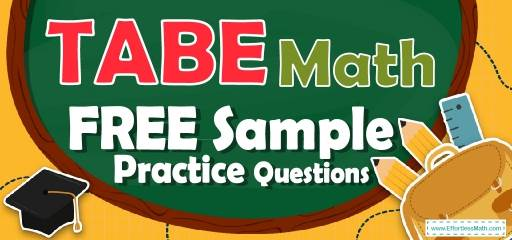# TABE Math FREE Sample Practice QuestionsPreparing for the TABE Math test? To do your best on the TABE Math test, you need to review and practice real TABE Math questions.  There’s nothing like working on TABE Math sample questions to hone your math skills and put you more at ease when taking the TABE Math test. The sample math questions you’ll find here are brief samples designed to give you the insights you need to be as prepared as possible for your TABE Math test.

Check out our sample TABE Math practice questions to find out what areas you need to practice more before taking the TABE Math test!

Start preparing for the 2022 TABE Math test with our free sample practice questions. Also, make sure to follow some of the related links at the bottom of this post to get a better idea of what kind of mathematics questions you need to practice.

## 10 Sample TABE Math Practice Questions

1- $$5 \frac{1}{2}+8 \frac{1}{3}=$$

A. $$3 \frac{5}{6}$$

B. $$13 \frac{6}{5}$$

C. $$13 \frac{5}{6}$$

D. $$3 \frac{6}{5}$$

2- $$6 \frac{2}{5}-2 \frac{1}{5}=$$

A. $$4 \frac{1}{5}$$

B. $$3 \frac{1}{5}$$

C. $$7 \frac{1}{5}$$

D. $$12 \frac{1}{5}$$

3- $$5\frac{2}{3} -\frac{3}{4}=$$

A. $$5\frac{1}{12}$$

B. $$4\frac{1}{12}$$

C. $$4\frac{11}{12}$$

D. $$4\frac{6}{12}$$

4- $$\frac{2}{3} ×\frac{4}{5}=$$

A. $$\frac{2}{5}$$

B. $$\frac{4}{15}$$

C. $$\frac{8}{15}$$

D. $$\frac{4}{5}$$

5- $$\frac{4}{5}÷\frac{1}{10}=$$

A. $$\frac{4}{50}$$

B. $$\frac{5}{50}$$

C. $$\frac{1}{40}$$

D. 8

6- $$2\frac{2}{3}×2 \frac{1}{6}=$$

A. $$2 \frac{7}{9}$$

B. $$5 \frac{9}{7}$$

C. $$5 \frac{7}{9}$$

D. $$2 \frac{9}{7}$$

7- $$12ab-5ab=$$

A. 17ab

B. 7ab

C. 6ab

D. 16ab

8- $$4^3×4^5=$$

A. $$4^{15}$$

B. $$4^{8}$$

C. $$4^{2}$$

D. $$4^{5}$$

9- $$4 \frac{3}{5} ÷2\frac{6}{10} =$$

A. $$1 \frac{10}{13}$$

B. $$1 \frac{13}{10}$$

C. $$\frac{10}{13}$$

D. $$2 \frac{13}{10}$$

10- $$5\%$$ of $$20=$$

A. 5

B. 4

C. 3

D. 1

## Best TABE Math Prep Resource for 2022

1- C
$$5 \frac{1}{2}+8 \frac{1}{3}=13 \frac{5}{6}$$

2- A
$$6 \frac{2}{5}-2 \frac{1}{5}= 6 + \frac{2}{5} -2-\frac{1}{5}=4 \frac{1}{5}$$

3- C
$$5\frac{2}{3} -\frac{3}{4}=4 \frac{11}{12}$$

4- C
$$\frac{2}{3} ×\frac{4}{5}=\frac{8}{15}$$

5- D
$$\frac{4}{5}÷\frac{1}{10}= 8$$

6- C
$$2\frac{2}{3}×2 \frac{1}{6}= \frac{8}{3} ×\frac{13}{6}=\frac{104}{18}=5 \frac{7}{9}$$

7- B
$$12ab – 5ab=7ab$$

8- B
$$4^3×4^5=4^{5+3}= 4^8$$

9- A
$$4 \frac{3}{5} ÷2\frac{6}{10} =\frac{23}{5} ÷\frac{13}{10}= \frac{115}{65}=1 \frac{10}{13}$$

10- D
$$5\% \space of \space 20 = 0.05 × 20 = 1$$

Looking for the best resource to help you succeed on the TABE Math test?

## More from Effortless Math for TABE Test …

### Repetitive learning methods of TABE test math has become boring for you?

Then you can use the top math websites to learn: Top 10 Free Websites for TABE Math Preparation.

### Tired of learning the TABE math test formulas separately and sparsely?

We provide you with a list of organized formulas: TABE Math Formulas.

### Do not have enough practice resources to prepare for the TABE math test?

Use our great worksheets: TABE Math Worksheets: FREE & Printable.

## Have any questions about the TABE Test?

### What people say about "TABE Math FREE Sample Practice Questions - Effortless Math: We Help Students Learn to LOVE Mathematics"?

No one replied yet.

X
52% OFF

Limited time only!

Save Over 52%

SAVE $40 It was$76.99 now it is \$36.99